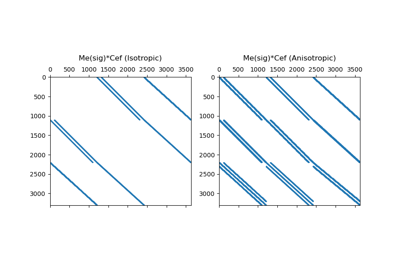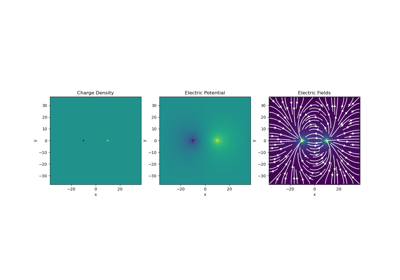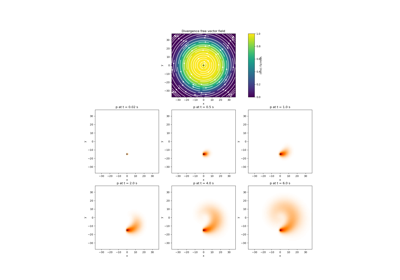# discretize.operators.DiffOperators#class discretize.operators.DiffOperators[source]#

Class used for creating differential and averaging operators.

DiffOperators is a class for managing the construction of differential and averaging operators at the highest level. The DiffOperator class is inherited by every discretize mesh class. In practice, differential and averaging operators are not constructed by creating instances of DiffOperators. Instead, the operators are constructed (and sometimes stored) when called as a property of the mesh.

Attributes

 average_edge_x_to_cell Averaging operator from x-edges to cell centers (scalar quantities). average_edge_y_to_cell Averaging operator from y-edges to cell centers (scalar quantities). average_edge_z_to_cell Averaging operator from z-edges to cell centers (scalar quantities). average_face_x_to_cell Averaging operator from x-faces to cell centers (scalar quantities). average_face_y_to_cell Averaging operator from y-faces to cell centers (scalar quantities). average_face_z_to_cell Averaging operator from z-faces to cell centers (scalar quantities). boundary_edge_vector_integral Represents the operation of integrating a vector function on the boundary boundary_node_vector_integral Represents the operation of integrating a vector function dotted with the boundary normal cellGrad cellGrad has been deprecated. cellGradBC cellGradBC has been deprecated. cellGradx cellGradx has been deprecated. cellGrady cellGrady has been deprecated. cellGradz cellGradz has been deprecated. cell_gradient Cell gradient operator (cell centers to faces) cell_gradient_BC Boundary conditions matrix for the cell gradient operator (Deprecated) cell_gradient_x X-derivative operator (cell centers to x-faces) cell_gradient_y Y-derivative operator (cell centers to y-faces) cell_gradient_z Z-derivative operator (cell centers to z-faces) faceDivx faceDivx has been deprecated. faceDivy faceDivy has been deprecated. faceDivz faceDivz has been deprecated. face_x_divergence X-derivative operator (x-faces to cell-centres) face_y_divergence Y-derivative operator (y-faces to cell-centres) face_z_divergence Z-derivative operator (z-faces to cell-centres) stencil_cell_gradient_x Differencing operator along x-direction (cell centers to x-faces) stencil_cell_gradient_y Differencing operator along y-direction (cell centers to y-faces) stencil_cell_gradient_z Differencing operator along z-direction (cell centers to z-faces)
 average_cell_to_edge average_cell_to_face average_cell_vector_to_face average_edge_to_cell average_edge_to_cell_vector average_edge_to_face_vector average_face_to_cell average_face_to_cell_vector average_node_to_cell average_node_to_edge average_node_to_face boundary_face_scalar_integral edge_curl face_divergence nodal_gradient nodal_laplacian project_edge_to_boundary_edge project_face_to_boundary_face project_node_to_boundary_node stencil_cell_gradient

Methods

 cell_gradient_weak_form_robin([alpha, beta, ...]) Robin conditions for weak form of the cell gradient operator (cell centers to faces) edge_divergence_weak_form_robin([alpha, ...]) Robin conditions for weak form of the edge divergence operator (edges to nodes) getBCProjWF(*args, **kwargs) getBCProjWF has been deprecated. getBCProjWF_simple(*args, **kwargs) getBCProjWF_simple has been deprecated. get_BC_projections(BC[, discretization]) The weak form boundary condition projection matrices. get_BC_projections_simple([discretization]) The weak form boundary condition projection matrices when mixed boundary condition is used setCellGradBC(*args, **kwargs) setCellGradBC has been deprecated. Set zero Dirichlet/Neumann boundary conditions for derivative operators acting on cell-centered quantities.

## Galleries and Tutorials using discretize.operators.DiffOperators#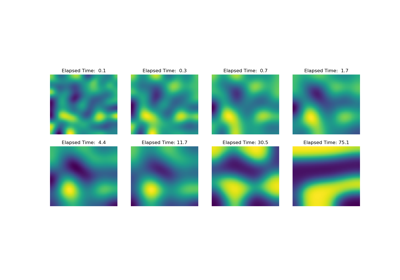Operators: Cahn Hilliard

Operators: Cahn Hilliard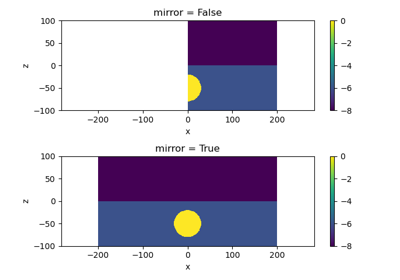Plot Mirrored Cylindrically Symmetric Model

Plot Mirrored Cylindrically Symmetric Model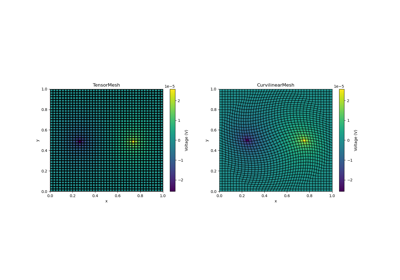Basic Forward 2D DC Resistivity

Basic Forward 2D DC ResistivityBasic: PlotImage

Basic: PlotImage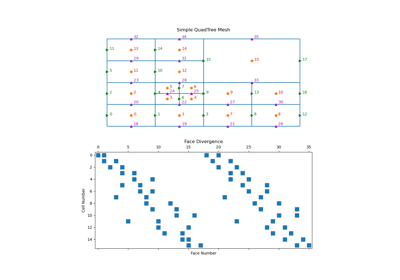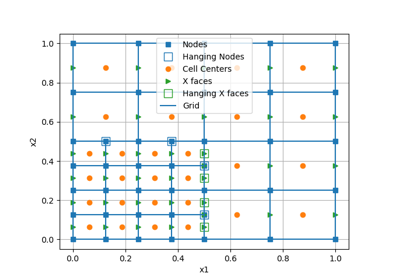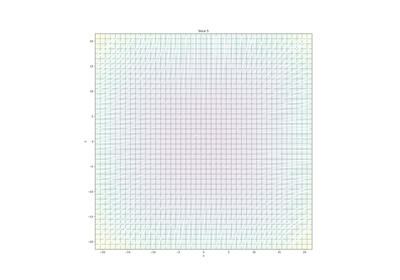Plotting: Streamline thickness

Plotting: Streamline thickness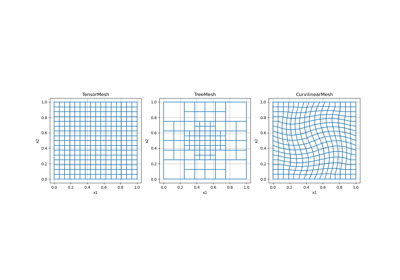Overview of Mesh Types

Overview of Mesh Types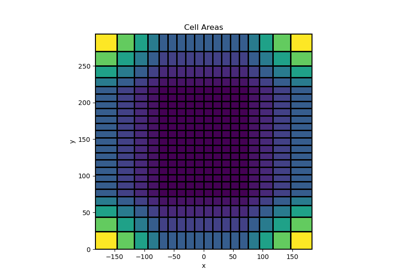Tensor meshes

Tensor meshes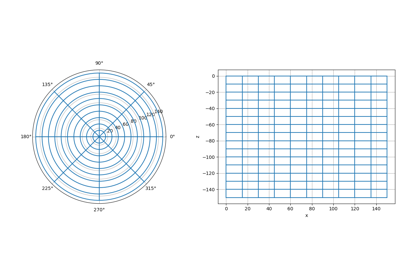Cylindrical meshes

Cylindrical meshes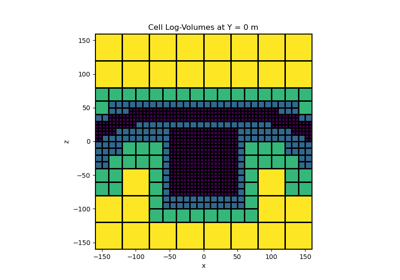Tree Meshes

Tree Meshes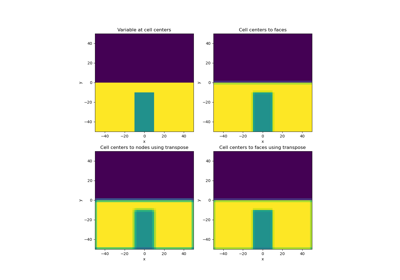Averaging Matricies

Averaging Matricies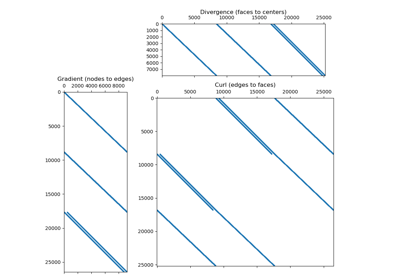Differential Operators

Differential Operators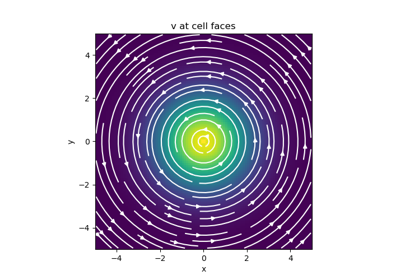Basic Inner Products

Basic Inner Products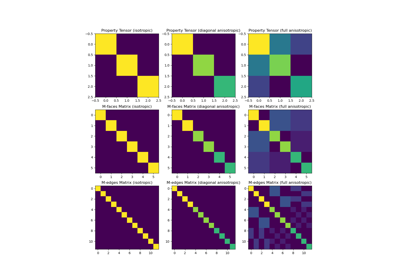Constitutive Relations

Constitutive Relations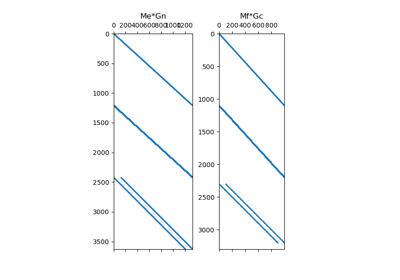Differential Operators

Differential Operators